×#### Thank you for registering.

One of our academic counsellors will contact you within 1 working day.

Click to Chat

1800-1023-196

+91-120-4616500

CART 0

• 0

MY CART (5)

Use Coupon: CART20 and get 20% off on all online Study Material

ITEM
DETAILS
MRP
DISCOUNT
FINAL PRICE
Total Price: Rs.

There are no items in this cart.
Continue Shopping• Complete JEE Main/Advanced Course and Test Series
• OFFERED PRICE: Rs. 15,900
• View Details

```Chapter 4: Cubes and Cube Roots Exercise – 4.4

Question: 1

Find the cube roots of each of the following integers:

(i) – 125

(ii) – 5832

(iii) – 2744000

(iv) – 753571

(v) – 32768

Solution: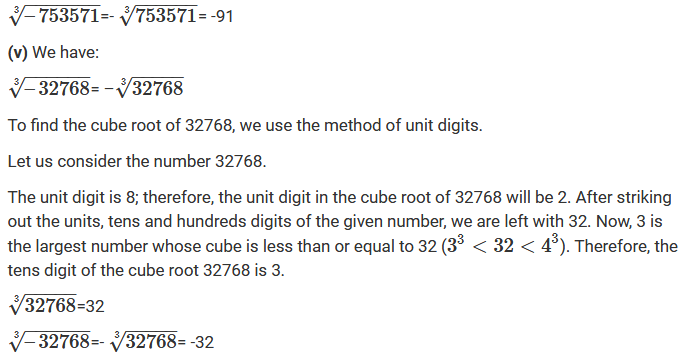Question: 2

Show that: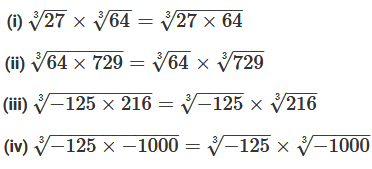Solution: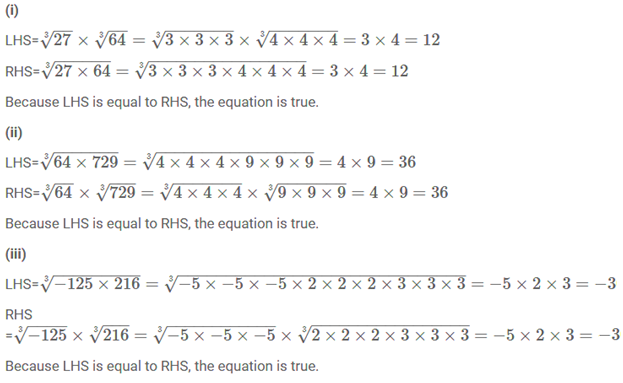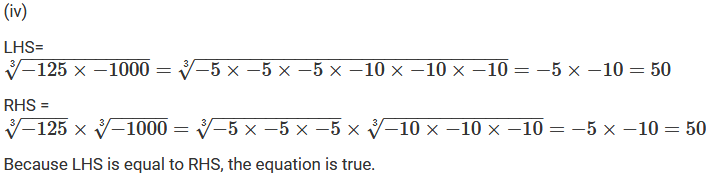Question: 3

Find the cube root of the following numbers:

(i) 8 × 125

(ii) −1728 × 216

(iii) −27 × 2744

(iv) − 729 × −15625

Solution: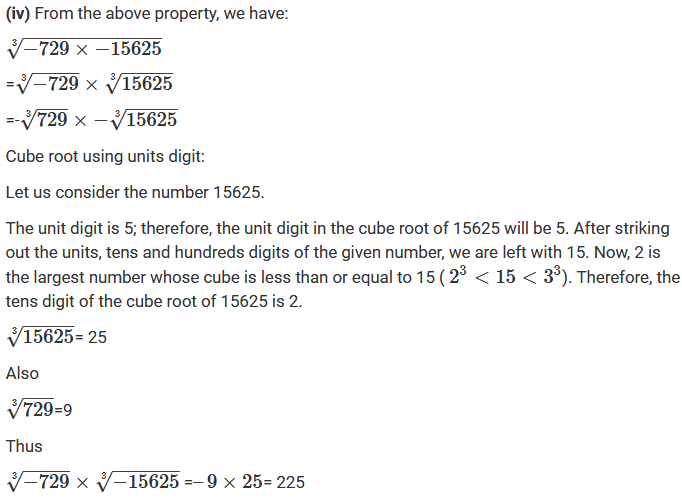Question: 4

Evaluate:Solution: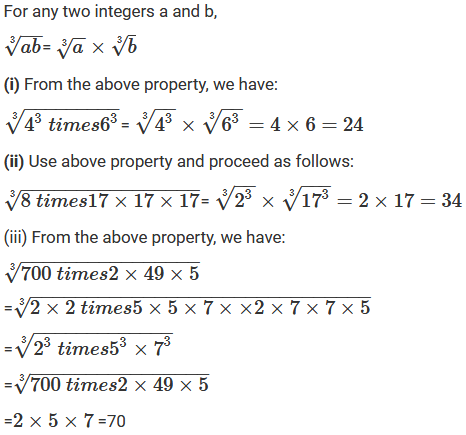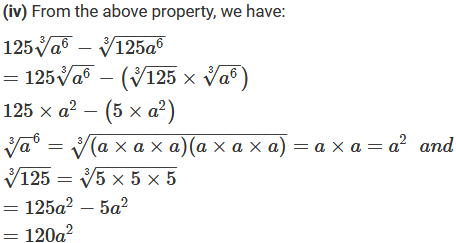Question: 5

Find the cube root of each of the following rational numbers: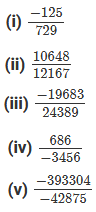Solution: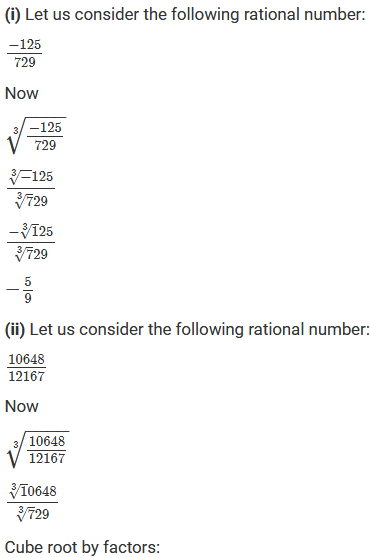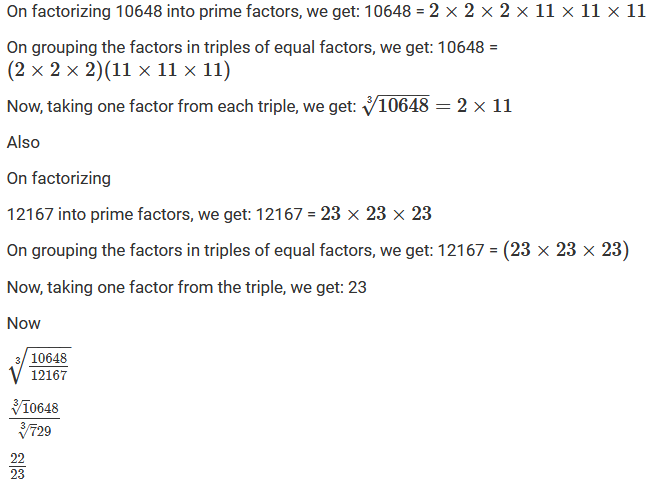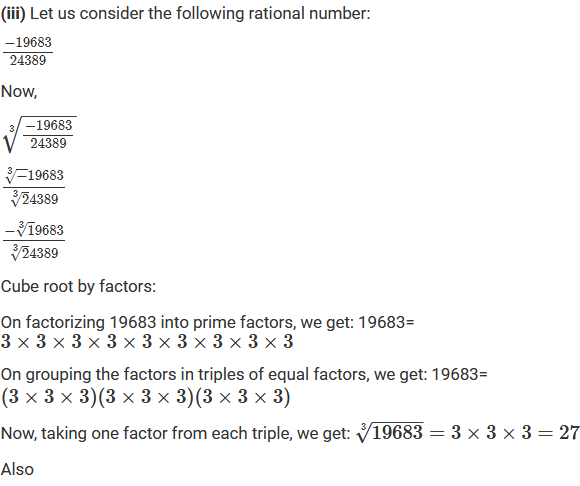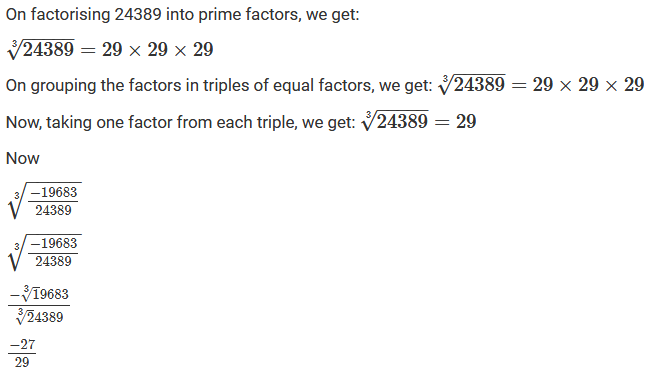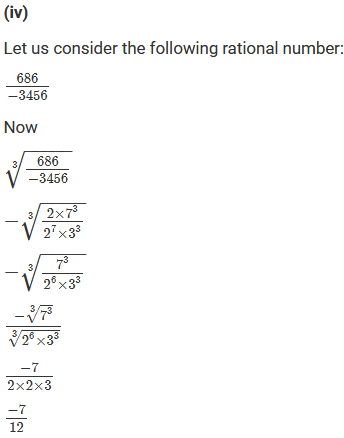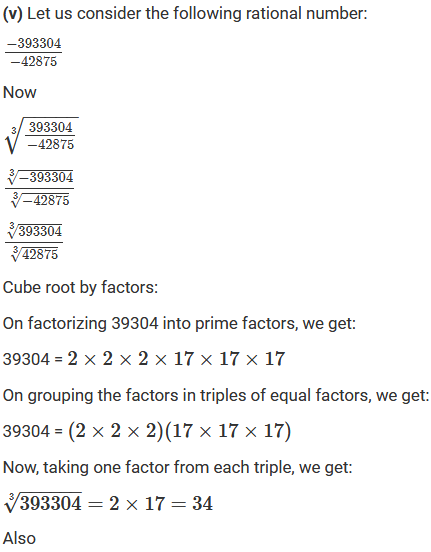Question: 6

Find the cube root of each of the following rational numbers

(i) 0.001728

(ii) 0.003375

(iii) 0.001

(iv) 1.331

Solution: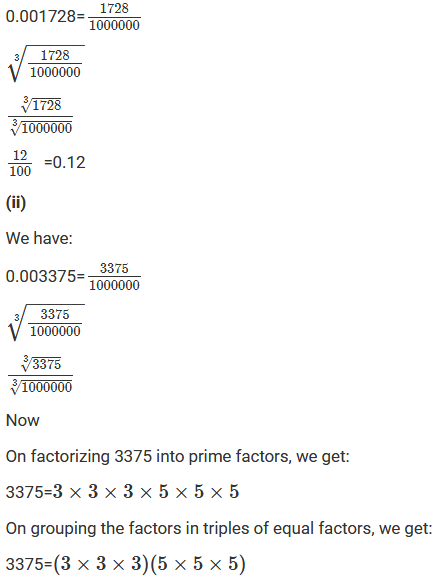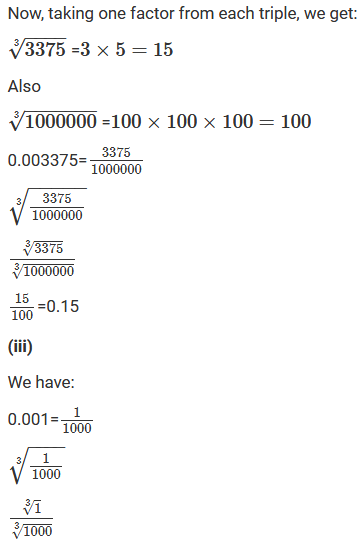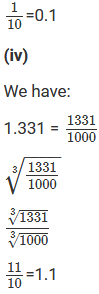Question: 7

Evaluate each of the following: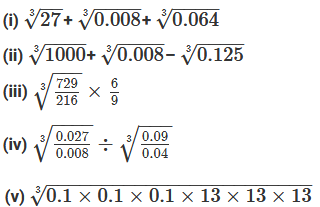Solution: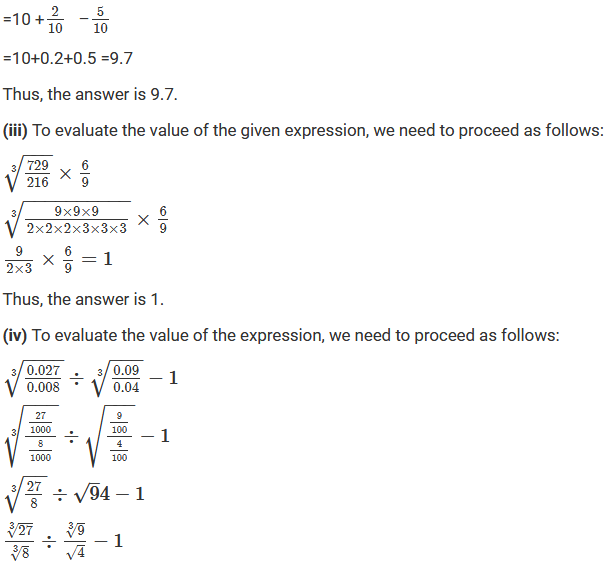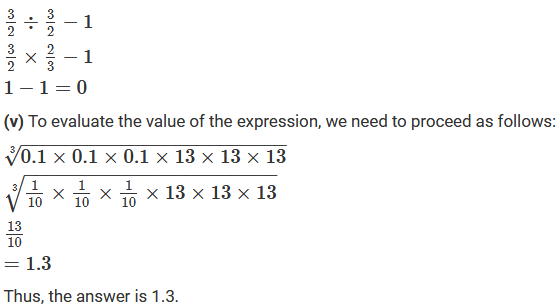Question: 8

Fill in the blanks:Solution:Question: 9

The volume of a cubical box is 474.552 cubic meters. Find the length of each side of the box.

Solution: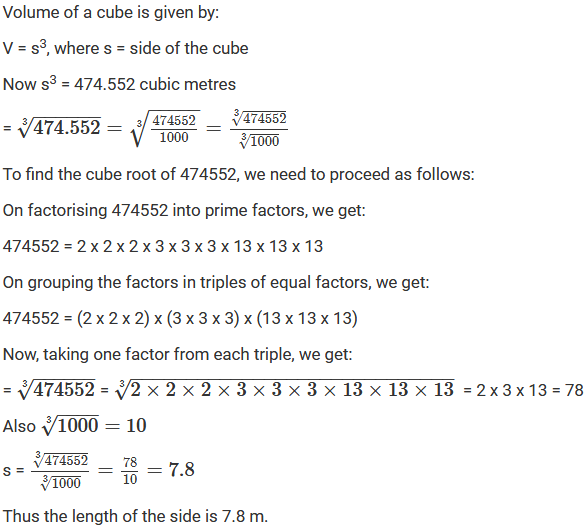Question: 10

Three numbers are to one another 2: 3: 4. The sum of their cubes is 0.334125. Find the length of each side of the box.

Solution: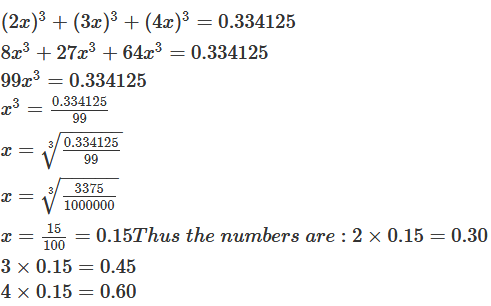Question: 11

Three numbers are to one another 2: 3: 4. The sum of their cubes is 0.334125. Find the length of each side of the box.

Solution: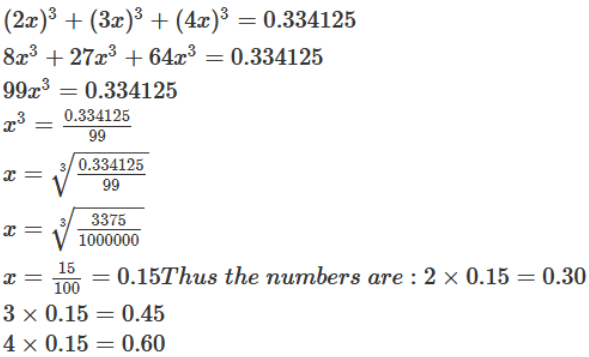Question: 12

Find the side of a cube whose volume is 24389/216 m3.

Solution:Question: 13

Evaluate: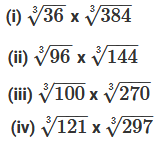Solution: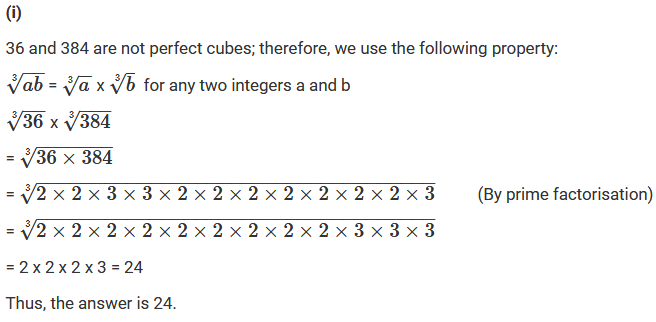Question: 14

Find the cube roots of the numbers 3048625, 20346417, 210644875, 57066625 using the fact that:

(i) 3048625 = 3375 x 729

(ii) 20346417 = 9261 x 2197

(iii) 210644875 = 42875 x 4913

(iv) 57066625 = 166375 x 343

Solution: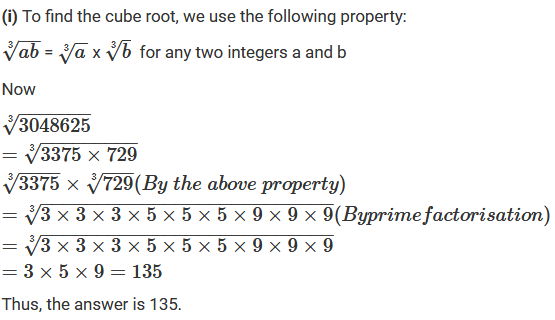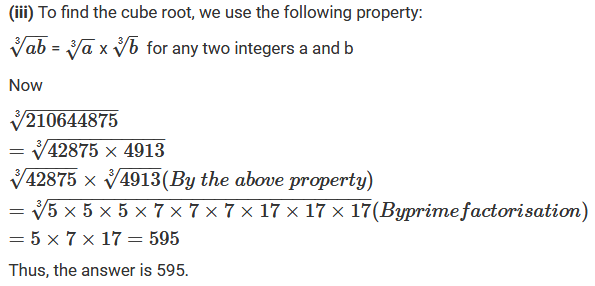Question: 14

Find the units digit of the cube root of the following numbers:

(i) 226981

(ii) 13824

(iii) 571787

(iv) 175616

Solution:

(i) Cube root using units digit:

Let us consider the number 226981. The unit digit is 1; therefore, the unit digit of the cube root of 226981 is 1.

(ii) Cube root using units digit:

Let us consider the number 13824. The unit digit is 4; therefore, the unit digit of the cube root of 13824 is 4.

(iii) Cube root using units digit:

Let us consider the number 571787. The unit digit is 7; therefore, the unit digit of the cube root of 571787 is 3.

(iv) Cube root using units digit:

Let us consider the number 175616. The unit digit is 6; therefore, the unit digit of the cube root of 175616 is 6.

Question: 15

Find the tens digit of the cube root of the each of the numbers in Q. No. 14.

Solution:

(i) Let us consider the number 226981.

The unit digit is 1; therefore, the unit digit of the cube root of 226981 is 1.

After striking out the units, tens and hundreds of digits of the given number, we are left with 226.

Now, 6 is the largest number, whose cube is less than or equal to 226 (63 < 226 < 73).

Therefore, the tens digit of the cube root of 226981 is 6.

(ii) Let us consider the number 13824.

The unit digit is 4; therefore, the unit digit of the cube root of 13824 is 4.

striking out the units, tens and hundreds of digits of the given number, we are left with 13.

Now, 2 is the largest number, whose cube is less than or equal to 13 (23 < 13 < 33).

Therefore, the tens digit of the cube root of 13824 is 2.

(iii) Let us consider the number 571787.

The unit digit is 7; therefore, the unit digit of the cube root of 571787 is 3.

After striking out the units, tens and hundreds of digits of the given number, we are left with 571.

Now, 8 is the largest number, whose cube is less than or equal to 571 (83 < 571 < 93).

Therefore, the tens digit of the cube root of 571787 is 8.

(iv) Let us consider the number 175616.

The unit digit is 6; therefore, the unit digit of the cube root of 175616 is 6.

After striking out the units, tens and hundreds of digits of the given number, we are left with 175.

Now, 5 is the largest number, whose cube is less than or equal to 175 (53 < 175 < 63).

Therefore, the tens digit of the cube root of 175616 is 5.
```### Course Features

• 728 Video Lectures
• Revision Notes
• Previous Year Papers
• Mind Map
• Study Planner
• NCERT Solutions
• Discussion Forum
• Test paper with Video Solution